(Difference between revisions)
Tohline (Talk | contribs)
(Begin separate chapter discussion Staher's (1983) mass-radius relationship for n=5 embedded polytropes)

Stahler's Mass-Radius Relationship for Embedded Polytropes

In an accompanying chapter that discusses detailed force-balanced models of embedded (and pressure-truncated) polytropes, we review S. W. Stahler's (1983) pair of parametric relations for the equilibrium mass and equilibrium radius for such systems, namely,$~M$$~=~$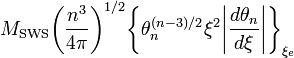$M_\mathrm{SWS} \biggl( \frac{n^3}{4\pi} \biggr)^{1/2} \biggl\{ \theta_n^{(n-3)/2} \xi^2 \biggl| \frac{d\theta_n}{d\xi} \biggr| \biggr\}_{\xi_e}$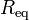$~R_\mathrm{eq}$$~=~$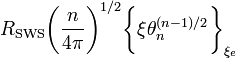$R_\mathrm{SWS} \biggl( \frac{n}{4\pi} \biggr)^{1/2} \biggl\{ \xi \theta_n^{(n-1)/2} \biggr\}_{\xi_e}$

where,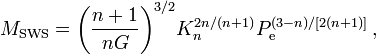$M_\mathrm{SWS} = \biggl( \frac{n+1}{nG} \biggr)^{3/2} K_n^{2n/(n+1)} P_\mathrm{e}^{(3-n)/[2(n+1)]} \, ,$$R_\mathrm{SWS} = \biggl( \frac{n+1}{nG} \biggr)^{1/2} K_n^{n/(n+1)} P_\mathrm{e}^{(1-n)/[2(n+1)]} \, ,$

and point out that Stahler (1983) (see his equation B13) explicitly states that the relevant mass-radius relationship for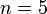$~n = 5$ embedded polytropes is,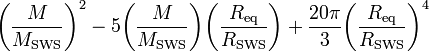$\biggl( \frac{M}{M_\mathrm{SWS}} \biggr)^2 - 5 \biggl( \frac{M}{M_\mathrm{SWS}} \biggr)\biggl( \frac{R_\mathrm{eq}}{R_\mathrm{SWS}} \biggr) + \frac{20\pi}{3} \biggl( \frac{R_\mathrm{eq}}{R_\mathrm{SWS}} \biggr)^4$$~=~$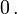$~0 \, .$

 © 2014 - 2021 by Joel E. Tohline |   H_Book Home   |   YouTube   | Appendices: | Equations | Variables | References | Ramblings | Images | myphys.lsu | ADS | Recommended citation:   Tohline, Joel E. (2021), The Structure, Stability, & Dynamics of Self-Gravitating Fluids, a (MediaWiki-based) Vistrails.org publication, https://www.vistrails.org/index.php/User:Tohline/citation